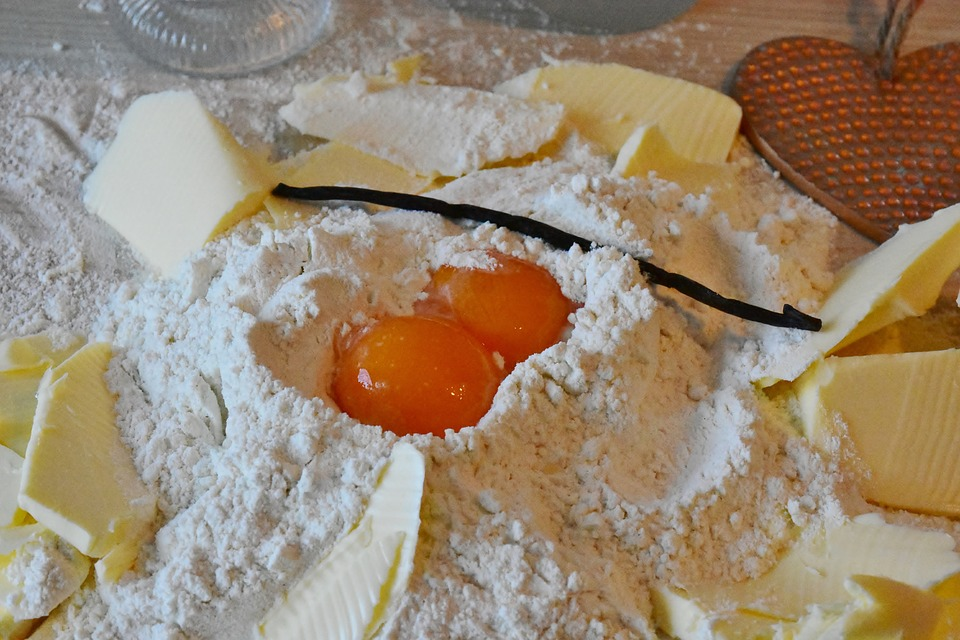# Difference Between How Many and How Much

The main difference between how many and how much is that how many refer to countable nouns whereas how much refers to uncountable nouns.

How many and how much are interrogative nouns. Hence. they are used to ask questions to know the number or quantity of certain things. It is necessary to know the difference between these two terms in order to understand their correct usage.

### Key Areas Covered

1. What Does How Many Mean
– Definition, Explanation, Examples
2. What Does How Much Mean
– Definition, Explanation, Examples
3. Similarity Between How Many and How Much
– Outline of Common Features
4. Difference Between How Many and How Much
– Comparison of Key Differences

### Key Terms

Grammar, English Language, How Many, How Much, Interrogative Nouns## What Does How Many Mean

By using how many, one questions the number of something. This essentially refers to something countable. Therefore, the question term how many requests for an answer with something countable as the answer.

Refer the given example sentences:

How many marbles do you have? (marbles can be counted)

How many pets does he like to have? ( Here, the countable number of pets is expected as the answer)

How many apples did I give you now?

How many siblings do you have?Figure 1: How many cupcakes are there?

How many words should be there for tomorrow’s essay?

How many coins did he get? (Here it is the number of coins that should be answered not the monetary value of them)

## What Does How Much Mean

One uses the interrogative term how much to know the amount of something uncountable. Uncountable means things that you can not count. Thus, How much is always preceded by an uncountable noun. Therefore, we can use this term with regard to uncountable references such as the amount of money, liquid quantities, food quantities, etc.

Refer the following example sentences;

How much money did you spend on this?

How much time did you spend reading this novel?

How much milk do these animals consume daily?

How much sugar do you need in your tea?

Moreover, when referring to quantities, these uncountable nouns are usually considered singular. However, when referring to price, it can be both singular or plural.

Quantity (singular)

How much tea is in the flask?

How much time is left for us?Figure 2: How much flour is needed to make this cake?

Price (Singular or Plural)

How much is this bag?

How much does this bracelet cost?

How much are those shoes?

How much do these paintings cost?

## Similarity Between How Many and How Much

• We can use both these interrogative terms to know the quantity or the amount of something we are referring to.

## Difference Between How Many and How Much

### Definition

How Many is an interrogative term used with countable nouns while How much is an interrogative term used with uncountable nouns.

### Usage

How many precede countable nouns such as the number of people, number of days, number of apples, number of bottles, number of people, number of books, etc. In contrast, how much precede uncountable nouns such as the amount of money, price and uncountable quantities such as time, liquid quantities, food quantities, etc.

### Conclusion

People often use the two interrogative nouns, how many and how much interchangeably; therefore, it is necessary to know the difference they have pertaining to their grammar and their respective usage. How many refer to countable nouns whereas how much refers to uncountable nouns. This is the basic difference between How Many and How Much.

##### Image Courtesy:

1. “Party Cupcakes Food Sweet Cake Dessert” (CC0) via Maxpixel
2. “Butter Flour Advent Egg Cake Bake Sugar Dough”(CC0) via Maxpixel